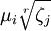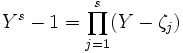## Friday, January 04, 2008

### Roots of Unity: Expressed as Radicals

In a previous blog, I showed how the roots of unity can be stated as trigonometric functions. The question remains about when and under what conditions, they can be expressed as radicals. After all, this is clearly the case if n is a power of 2.

Abraham de Moivre made significant progress in investigating this question. In today's blog, I will show that we can answer the case of a composite number. If n is a nonprime such that n=rs and the r-th roots of unity can be expressed as radicals and the s-th roots of unity can be expressed as radicals, then it follows that the n-th root of unity can be expressed as radicals.

Lemma 1:

Let r,s be positive integers
Let μ1, μ2, ..., μr be the r-th roots of unity.
Let ζ1, ζ2, ..., ζs be the s-th roots of unity.

Then:

The rs-th roots of unity are of the form:for i = 1, ..., r and j = 1, ..., s.

Proof:

(1) Using an earlier result (see Lemma 1, here), we have:(2) Setting Y = Xr gives us:(3) Using de Moivre's equation (see Theorem 1, here), we know that:(4) Combining step #2 and step #3, we have:QED

Theorem 2:

Let n be a positive integer.

If for each prime p factor of n, the p-th root of unity is expressible as radicals, then the n-th root of unity is expressible as radicals

Proof

(1) If n is a prime number, then the theorem is true by definition. So, for n=2, this theorem holds.

(2) Assume that there exists some number n such that the theorem holds true for all numbers less than n.

(3) If n is a prime, then the theorem is true and we are done. So, we assume that n is not a prime.

(4) Then there exists two numbers r,s such that r≠ 1 and s≠1 and n =rs.

(5) Since by assumption the r-th roots of unity and the s-th roots of unity are expressible by radicals, we can apply Lemma 1 above to conclude that n-th roots of unity must also be expressible by radicals.

QED

The implication of Theorem 2 is that to determine which n-th roots of unity are expressible as radicals, we only need to consider the cases where n is a prime number.

References